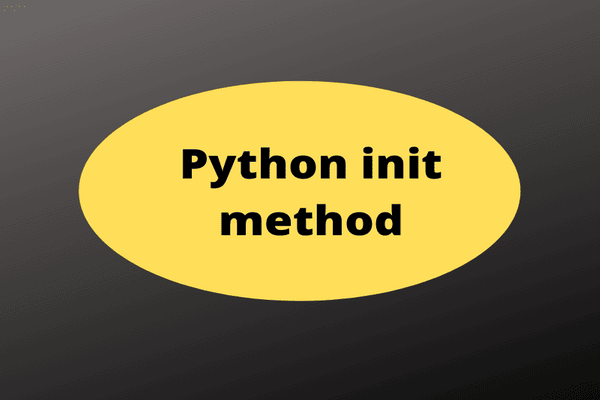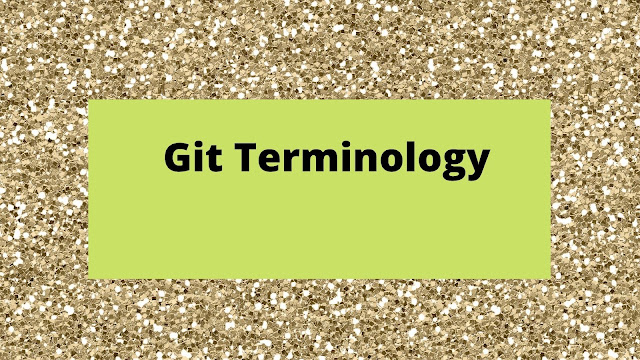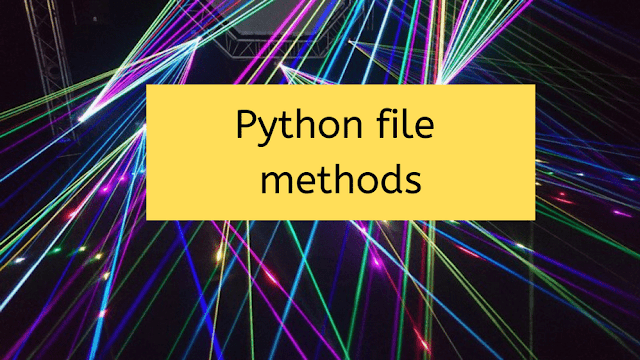Posts

How to write init Class method in PythonHere is a Python sample class that uses the init method. The init method is like a constructor in Java. But the usage is different in Python. So init method is the first method in the Python class with all the initializations. The dog_1 is an instantiation of dog class. When you print the dog_1, it prints as I am in the init method from the init method. The self means this class. It is mandatory. Not only self, but you can also give any name in that place. Instated of 'self' you can give as This_is_Python_Self . Then also it will work. Class with init method class dog:    def __init__(self):          print("I am in init method") Now, instantiating the class dog_1=dog() print(dog_1) The output from the above display is as below: I am in init method <__main__.dog object at 0x7fe7b5875be0> Related posts The best Python Interview Questions

How to Unpack Using Python Splat OperatorThe splat operator, in python, changes the format of the display of the output. The asterisk in python is called a spalt operator. There are two types of splat operators. Those are single and double. Here are the best examples of those. 1. Single splat operator Consider, for example, this code:  abc = [1,2,3,4]  print(abc) In this example, our output is: [1, 2, 3, 4] What if you didn't want the list output in list format? What if all you wanted was the list of values to be written to the output console? You could write them using a loop and one of the output functions, but Python prefers an easier way: print(*abc) 1 2 3 4 2. Double splat operator Here, I have written a function: def func(x,y,z):        return x + y + z print(func(**d)) It will show '6' as output. Since, I have assigned values for x,y, and z in a dictionary. So by using a double splat operator you assign values to the function. d = {  'x': 1,  'y': 2,  'z': 3  } Related posts 3 Advanc

9 Top Git Terminology You needHere're top terminology popular in Git version control system. These are useful why means interviewer surely surface these questions. So if you can respond to these, interviewer will acknowledge that you have enough subject knowledge. Git Vs GitHub This is the first question you ( even me also) might confuse. Git is version control system. Whereas GitHub is repository frame-work . Also you can say GitHub is Git hosting service. What is Branch Git is light-weight version control system. In simple terms, a Branch is a separate line of development. You can have any number of branches in the Git. What is Topic Each branch in the Git refers to a particular purpose. So topic tells about the purpose. Clone In easy terms, the Clone means copying a existing repository. So you can say it is just a copy of existing repository. What is Push You can say Push means updating the existing repository. In other words, developers push their changes to a repository that you setup. Merge Merge unifiesHow to Write Python Recursive FunctionRecursive functions act like loops that they iterate within the function to perform some operation.  Since you are calling the same function in the function definition, it's called recursive . The developer must follow some rules to write a recursive function.  Else, it will go into a loop. So developers must check twice the recursive function code. Four rules Here are the four top rules you need to consider, before you write a recursive function. There must be a key variable, which will be responsible for the termination of recursion. To determine the base value , which the key variable has to meet to reach the termination. To make sure the key variable must approach the base value in every recursive call. To make the recursive function terminate when the key variable reaches the base value. Python recursive function Here is a sample recursive function: # This program computes the factorial of a number using recursion #function definition def fact(n): "computes factorial us

How to Use Set Comprehension in PythonPython also allows for set comprehensions. As expected, you can't modify an existing set with a comprehension, only create a new one. Set comprehensions basically the purpose to create a new set Set Comprehension in Python Likewise, of course, the comprehension must result in a valid set. A set cannot contain multiple entries of the same value . Like the dictionary, Python is polite about this.  If you try to add values to the set that are already there, it will replace the old one with the new one. Curling brackets for set comprehension Set comprehensions using the {} syntax only exist in Python 3. Before that, you'll have to use the set() function to create and work with sets. You might guess, therefore, that one of the best uses of a set is to eliminate duplicates.  In fact, this is one of the most basic forms of set comprehension. Given a list, we can duplicate it as a list with a simple list comprehension like this: List logic list_copy = [x for x in original_list] It sho

Sets Vs Lists Python Programmer TipsSets are only useful when trying to ensure unique items are preserved. Before sets were available, it was common to process items and check if they exist in a list (or dictionary) before adding them. List example Here unique is an empty list. Every time I compare with this list, and if it is not duplicated then the input item will append to the unique list.  >>> unique = []  >>> for name in ['srini', 'srini', 'rao', 'srini']:  ... if name not in unique:  ... unique.append(name)  ... >>> unique ['srini', 'rao'] There is no need to do this when using sets. Instead of appending you add to a set: Set example >>> for name in ['srini', 'srini', 'rao', 'srini']: ... unique.add(name)  ...  >>> unique {'srini', 'rao'} Just like tuples and lists, interacting with sets have some differences on how to access their items. You can't index them like lists an